## Solving Rational Inequalities AnalyticallyThis section assumes that you know how to solve polynomial inequalities analytically.

A rational function is a quotient of two polynomials. Let's look at the inequality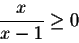as an example for an inequality involving a rational function. Here is the graph of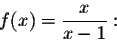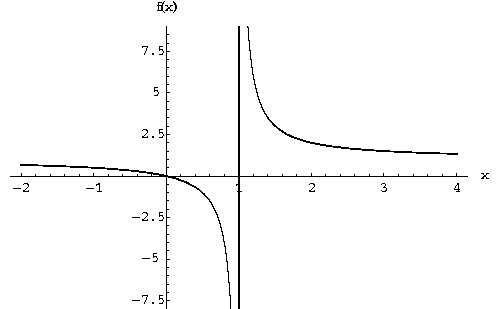The crucial question we have to answer to solve our inequality is: Where can the function f(x) change its sign?

As in the case of polynomials, f(x) can change its sign, where f(x)=0. (In our case this happens when x=0.) Note that f(x)=0 exactly if the numerator of f(x) equals 0.

But as you can see, the function f(x) also changes its sign rather dramatically at x=1. What happens at x=1? The denominator of f(x) equals 0 at x=1. In particular, f(1) is undefined, since one cannot divide by 0. In almost all cases, this means that the graph of f(x) has a vertical asymptote at points where the denominator is equal to 0. At a vertical asymptote the sign of f(x) might change, from "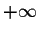" to "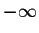", or vice versa.

This suggests the following method to solve rational inequalities:

Step 1. Find all points x where the numerator of f(x) equals 0, and find all points x where the denominator of f(x) equals 0. Draw a picture of the x-axis and mark these points. (I will indicate the points where the numerator is 0 by yellow dots, and the points where the denominator equals 0 by green dots. Your teacher might call this collection the critical points of the inequality.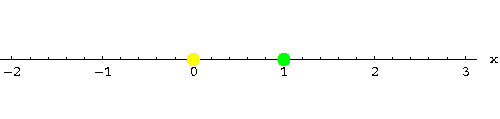Step 2. The rest of the procedure is more or less identical to the one we used for polynomial inequalities. Our critical points partition the x-axis into three intervals. Pick a point (your choice!) in each interval. Let me take x=-1, x=1/2 and x=2. Compute f(x) for these points: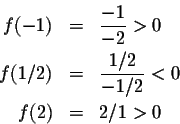These three points are representative for what happens in the intervals they are contained in:

Since f(-1)>0, f(x) will be positive for all x in the interval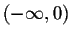. Similarly, since f(1/2)<0, f(x) will be negative for all x in the interval (0,1). Since f(2)>0, f(x) will be positive for all x in the interval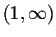. You can indicate this on the x-axis by inserting plus or minus signs on the x-axis. I use color coding instead: blue for positive, red for negative: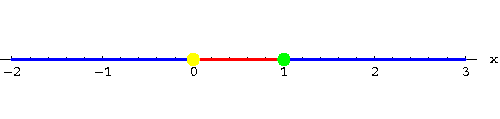Step 3. We want to solve the inequality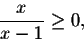so we are looking for all x such that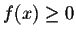. Consequently, the set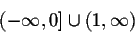contains all solutions to the inequality. Since f(0)=0, x=0 is a solution of the inequality, so we include it into the set of solutions. x=1, on the other hand, is not a solution. You can't even plug x=1 into f(x) to check whether it is a solution. "Green dots" (denominator equals 0) will never be part of the set of solutions.

Warning! I know that mathematicians make everything complicated, but the shortcut you might think of leads to incorrect answers. We wanted to solve the inequality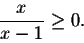Why don't we just multiply both sides by the denominator x-1 to obtain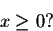(Note that this answer is not correct!) We can only multiply an inequality by a number if we know its sign. Do you remember why? But a second ago we multiplied by x-1. Whether x-1 is positive or negative depends on the unknown variable x.

Here is another example: Find the solutions of the inequality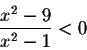Step 1. The numerator equals 0, when x=-3 and when x=3. The denominator equals 0, when x=-1 and when x=1. Draw a picture of the x-axis and mark these points. I will indicate the points where the numerator is 0 by yellow dots, and the points where the denominator equals 0 by green dots.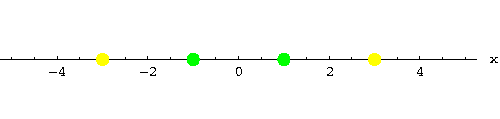Step 2. Our critical points partition the x-axis into five intervals. Pick a point (your choice!) in each interval. Let me take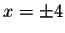,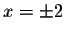and x=0. Compute f(x) for these points: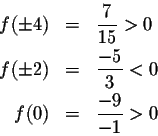These five points are representative for what happens in the intervals they are contained in:

Since f(-4)>0, f(x) will be positive for all x in the interval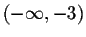. Similarly, since f(-2)<0, f(x) will be negative for all x in the interval (-3,-1). Since f(0)>0, f(x) will be positive for all x in the interval (-1,1). Since f(2)<0, f(x) will be negative for all x in the interval (1,3). Since f(4)>0, f(x) will be positive for all x in the interval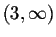. You can indicate this on the x-axis by inserting plus or minus signs on the x-axis. I use color coding instead: blue for positive, red for negative: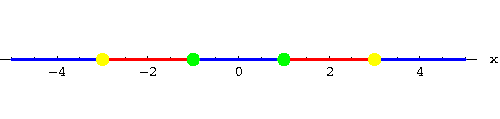Step 3. We want to solve the inequality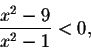so we are looking for all x such that f(x)< 0. Consequently, the set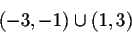contains all solutions to the inequality. Neither of the interval endpoints will be included, since our inequality was "strict".

(Did you notice that our function on the left side of the inequality is an even function? Consequently, the set of solutions will be symmetric with respect to x=0.)

Here is our next example: Find the solutions of the inequality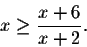For our method to work it is essential that one side of the inequality equals zero! So let's change our inequality to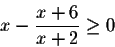Next we combine the terms on the left: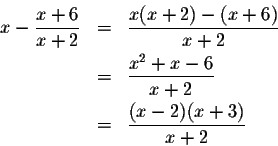Step 1. I already factored the numerator to make it easy to see that the numerator will be 0, when x=-3 and when x=2. The denominator vanishes at x=-2. Draw a picture of the x-axis and mark these points. I will indicate the points where the numerator is 0 by yellow dots, and the points where the denominator equals 0 by green dots.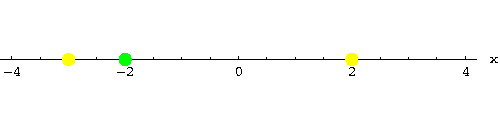Step 2. Our critical points partition the x-axis into four intervals. Pick a point (your choice!) in each interval. Let me take x=- 4, x=-2.5, x=0 and x=3. Compute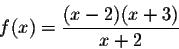for these points: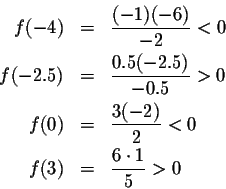These points are representative for what happens in the intervals they are contained in:

Since f(-4)<0, f(x) will be negative for all x in the interval. Similarly, since f(-2.5)>0, f(x) will be positive for all x in the interval (-3,-2). Since f(0)<0, f(x) will be negative for all x in the interval (-2,2). Since f(3)>0, f(x) will be positive for all x in the interval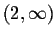. You can indicate this on the x-axis by inserting plus or minus signs on the x-axis. I use color coding instead: blue for positive, red for negative: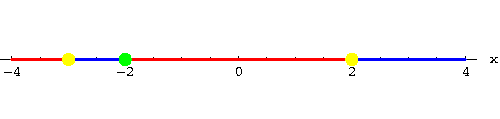Step 3. We want to solve the inequality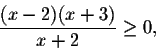so we are looking for all x such that. Consequently, the set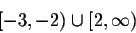contains all solutions to the inequality. Both x=-3 and x=2 are included since they make the numerator and thus f(x) equal to zero; x=-2 is excluded, since it makes the denominator of f(x) zero, and thus f(x) itself undefined.

Time for you to try it yourself:

#### Exercise 1.

Find the solutions of the inequality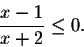#### Exercise 2.

Find the solutions of the inequality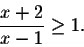#### Exercise 3.

Find the solutions of the inequality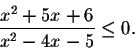#### Exercise 4.

Find the solutions of the inequality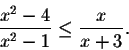#### Exercise 5.

Find the solutions of the inequality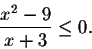[Back]
[Algebra] [Trigonometry] [Complex Variables]
[Calculus] [Differential Equations] [Matrix Algebra]S.O.S MATHematics home page

Do you need more help? Please post your question on our S.O.S. Mathematics CyberBoard.Helmut Knaust